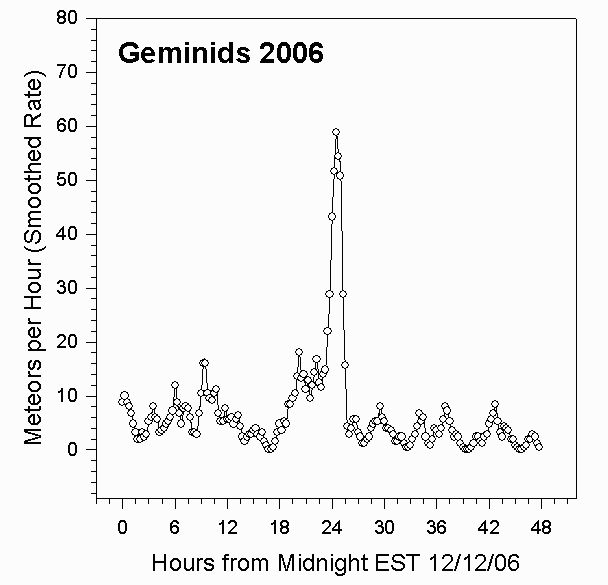Radio Observation of the 2006 Geminids

Data Collection and Analysis

For details about our equipment and method of data analysis, see our main page. The 2006 Geminid meteor shower showed one major peak of about 60 meteors per hour at 11:30 PM EST on December 13, 2006.

We've modified our data analysis. Instead of counting all meteors in an hour, we've counted meteors in quarter hour intervals, and then applied a data smoothing equation of the form

Smoothed_Rate(t) = 3.2*[((1/2)*N(t))+((1/4)*(N(t+1))+((1/4)*(N(t-1))+((1/8)*(N(t+2))+((1/8)*(N(t-2))]

where N(t) is the number of meteors in the fifteen minute interval t, N(t-1)is the number of meteors in the preceding fifteen minute interval, and so on.

Rates for 2006 Geminids

The following graph shows the meteor count rates per hour we measured for the 2006 Geminids. These rates were determined by a computer program that counted all peaks that climbed at least 20% above the background level in one second. The graph shows the rates observed for the 48 hour period starting at Midnight EST on 12/12/06.Summary

We observed one peak for the 2006 Geminid meteor shower. The peak rate of about 60 meteors per hour occurred at 11:30 PM EST on December 13, 2006.